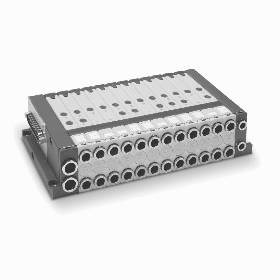Indonesia
English

## Valve Islands Series FMain features:
•It is a universal valve island that can be used in many different applications
•Flow rate: 250 Nl/min and 500 Nl/min (1000/1100 Nl/min for possible future sizes)
•Dimensions: 12 mm and 14 mm (18/19 mm are possible future sizes)
•Different valve functions
•Division of the valve island into pressure and vacuum zones
•Single modularity up to a maximum number of 24 coils.
•Multipole electrical connection

Code

 F P 2 R M T A - B2MULCA - 2QRSLRS

 `F` `Series` `P` ```Type: P = pneumatic A = accessories``` `2` ```Size: 1 = 12 mm 2 = 14 mm``` `R` ```Manual override: P = pressure actuation control R = actuation control with push & turn device``` `M` ```Electrical connection: M = multipole``` `T` ```Cartridges for left terminal: S = tube Ø 8 T = tube Ø 10``` `A` ```Type of servo-pilot: A = internal B = external Note: the cartdriges for the right terminal are for tube Ø 6.``` `-` `B2MULCA` ```Type of solenoid valves and additional plates *: M = 5/2 monostable B = 5/2 bistable C = 2x3/2 NC A = 2x3/2 NO G = 3/2 NC + 3/2 NO E = 2x2/2 NC F = 2x2/2 NO I = 2/2 NC + 2/2 NO V = 5/3 CC L = free position X = supplementary power supply and exhaust T = separated power supply and exhaust U = separated power supply, supplementary exhaust K = supplementary power supply, separated exhaust``` `-` `2QRSLRS` ```Cartridges for solenoid valves and additional plates *: Q = tube Ø 4 R = tube Ø 6 S = tube Ø 8 L = free position``` ``` * NOTE: in case of identical and consecutive codes, in the choices " type of solenoid valves and additional plates " and " cartridges for solenoid valves and additional plates ", letters have to be substituted with numbers. With the choice " cartridges for solenoid valves and additional plates " both connections (2 and 4) (1 and 3/5) are defined. Examples: FP2RMTA-MBCCMULMMMBB-QQRSSLRRRQRR FP2RMTA-MB2CMUL3M2B-2QR2SL3RQ2R ``` ``` CODING EXAMPLE OF A SOLENOID VALVE ``` `FP2V-MQR` `F` `Series` `P` ```Type: P = pneumatic``` `2` ```Size: 1 = 12 mm 2 = 14 mm``` `V` `Solenoid valve or additional plate` `-` `M` ```Type of function: M = 5/2 monostable B = 5/2 bistable C = 2 x 3/2 NC A = 2 x 3/2 NO G = 3/2 NC + 3/2 NO E = 2 x 2/2 NC F = 2 x 2/2 NO I = 2/2 NC + 2/2 NO V = 5/3 CC L = free position X = supplementary power supply and exhaust T = separated power supply and exhaust U = separated power supply and supplementary exhaust K = supplementary power supply and separated exhaust``` `Q` ```Cartridges dimensions: = no cartridge Q = Ø4 R = Ø6 S = Ø8 (not for size 1)``` `R` ```Type of manual override: R = push and turn (bistable) P = pressure (monostable)``` ``` CODING EXAMPLE OF A LEFT TERMINAL ``` ``` CODING EXAMPLE OF A RIGHT TERMINAL ``` `FA2T-S` `FA2T-AR` `F` `Series` `Series` `A` `Accessory` `Accessory` `2` ```Size: 1 = 12 mm 2 = 14 mm``` ```Size: 1 = 12 mm 2 = 14 mm``` `T` ```Type of accessory: T = left terminal``` ```Type of accessory: T = right terminal``` `-` `S` ```Cartridges: = no cartridge S = Ø8 T = Ø10``` ```Type of servo-pilot: A = internal B = external``` ```Cartridges: R = Ø6```